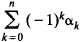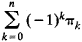# Euler Characteristic

Also found in: Wikipedia.
The following article is from The Great Soviet Encyclopedia (1979). It might be outdated or ideologically biased.

## Euler Characteristic

The Euler characteristic of a polyhedron is the number α0 — α1 + α2, where α0 is the number of vertices, α1 is the number of edges, and α2 is the number of faces. According to Euler’s theorem, if the polyhedron is convex or is homeomorphic to a convex polyhedron, then its Euler characteristic is 2. This fact was known to R. Descartes; L. Euler published a proof of the theorem in 1758.

The Euler characteristic of an arbitrary simplicial complex is the numberwhere n is the dimension of the complex, α0 is the number of vertices, and α1 is the number of edges. In general, k is the number of k-dimensional simplexes belonging to the complex. According to the Euler-Poincaré formula, the Euler characteristic is equal towhere πk is the k-dimensional Betti number of the complex. The topological invariance of the Euler characteristic follows from this fact.

In view of the topological invariance of the Euler characteristic, we speak of the Euler characteristic of a surface and of a polytope, by which we mean the Euler characteristic of any triangulation of the surface or polytope.

### REFERENCES

Aleksandrov, P. S. Kombinatornaia topologiia. Moscow-Leningrad, 1947.
Pontriagin, L. S. Osnovy kombinatornoi topologii, 2nd ed. Moscow, 1976.
References in periodicals archive ?
Spaces of PSL(2,C)-representatives of fundamental groups of surfaces of negative Euler characteristic are natural objects upon which mapping class groups and related automorphism groups act, they say, and arise as deformation spaces of (possibly singular) locally homogeneous geometric structures on surfaces.
We remark that these bounds hold trivially for the two closed surfaces with zero Euler characteristic; the torus and the Klein bottle.
Along the Euler characteristic approach, we choose as the nonlinear threshold in (3) the observed normalized Draupner crest height [xi] = 6.2 = 1.55 [H.sub.s]/[sigma] and derive the related probability.
This result is consistent with the mirror symmetry because the integral of the third Chern class [c.sub.3](E) for a vector bundle E is equal to the Euler characteristic of tangent bundle TM when E = TM and the third Chern class has a desired sign flip between a complex vector bundle E in the fundamental representation and its conjugate bundle [bar.E] in the antifundamental representation.
In this article we give the implementation of two algorithms to compute the Euler characteristic of Milnor fibre of reduced plane curve singularities in computer algebra system SINGULAR.
Equivalently, there are no normal surfaces with non-negative Euler characteristic, except for peripheral tori and Klein bottles.
The idea of enumeration using the Euler characteristic was suggested throughout Rota's work and influenced by Schanuel's categorical viewpoint [21, 23, 24, 25].
A useful homological interpretation (in terms of the Euler characteristic) of the Z-bilinear Tits form [[??].sub.J](x, y) = x x [[??].sub.J] x [y.sup.tr] (26)and Z-bilinear Euler form [[bar.b].sub.J](x, y) = x x [[bar.C].sub.J] x [y.sup.tr] is given in [1, (1.3)].
For d = 2 the intrinsic volumes are--up to constant factors--the area A = [V.sub.2], the boundary length L = 2[V.sub.1], and the Euler characteristic X = [V.sub.0], for d = 3 they are the volume V = [V.sub.3], the surface
Conway (George Washington U.) applies the Euler characteristic to map coloring, proves the spectral theorem for hermitian and normal linear transformations on a finite-dimensional Hilbert space, and develops the structure of a finitely generated torsion module.
One of the most famous of such tools is the Euler characteristic, which in its simplest version assigns a number to any polyhedron--a surface, such as that of a cube, made up of polygons that meet along lines and at corner points.

Site: Follow: Share:
Open / Close